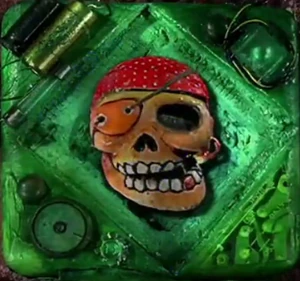## FANDOM

31 Pages

Captain Bones"Game time...Captain Bones!"

"*crazed laughter*"

## DescriptionEdit

Captain Bones is a moving object game starring a reanimated pirate skeleton who, out of boredom, makes visual math puzzles using his bones. At the start of each game, Captain Bones will collapse his bones and form a math problem, in the style of a digital clock or scoreboard, with them. The problem is always incorrect. So the goal is to fix the problem by only moving one or two bones.

Occasionally, Bones will instead form a picture with his bones, and the goal is to then change the picture into something else, also only moving one or two bones.

Throughout the game, Bones will openly mock and demean the player using several pirate-esque insults, eventually becoming so irate he demands you leave his sight - moving to the next game.

## Tips and TricksEdit

Honestly, I think you just have to rearrange every bone until you find the answer. This is most likely the hardest game being near Psycho Math's difficulty.

Have the solutions are straight up Bone-picking situations, good luck if you wanna hundred percent this.

## SegmentsEdit

Episode 2: 1 +1 = 6 to 7 -1 = 6 and 10 = 7 - 3 to 10 - 7 = 3

Episode 4: Fish to a tree and ice in a glass to not in the glass.

Episode 6: 1 + 1 = 6 to 7 - 1 = 6 and 7 - 6 = 12 to 7 + 5 = 12

Episode 7: Dog faces right to faces left and two houses to one house.

Episode 15: 1 + 1 = 6 to 7 - 1 = 6 and 10 - 8 = 18 to 18 - 8 = 10

Episode 16: 1 + 1 = 6 to 7 - 1 = 6 and 18 - 6 = 2 to 10 - 8 = 2

Episode 17: 1 2 3 4 5 6 7 (8) and O T T F F S S (E)

Episode 18: Pick a number 1 thru 10 then * 5 + 10 * 2 / 10 - # = 2

Episode 19: 1 + 1 = 6 to 7 - 1 = 6 and 7 + 3 = 9 to 7 + 2 = 9

Episode 23: Pick a number 1 thru 10 then * 2 + 4 / 2 - # = 2

Episode 24: 1 + 1 = 6 to 7 - 1 = 6 and 6 + 3 = 11 to 6 + 5 = 11

Episode 26: 1 + 1 = 6 to 7 - 1 = 6 and 1 + 2 = 8 to 7 + 2 = 9

Episode 28: Dog faces right to faces left and five squares to four squares.

Episode 30: 1 + 1 = 3 to 1 + 1 = 2 and 5 + 7 = 5 to 5 + 1 = 6

Episode 32: 1 + 1 = 3 to 1 + 1 = 2 and 16 + 7 = 17 to 10 + 7 = 17

Episode 34: Dog faces right to faces left and four squares to two squares

Episode 35: 1 + 1 = 3 to 1 + 1 = 2 and 9 + 6 = 3 to 3 + 6 = 9

Episode 37: 1 + 1 = 3 to 1 + 1 = 2 and 9 + 4 = 5 to 5 + 4 = 9

Episode 39: 1 + 1 = 3 to 1 + 1 = 2 and 5 + 9 = 11 to 5 + 6 = 11

Community content is available under CC-BY-SA unless otherwise noted.